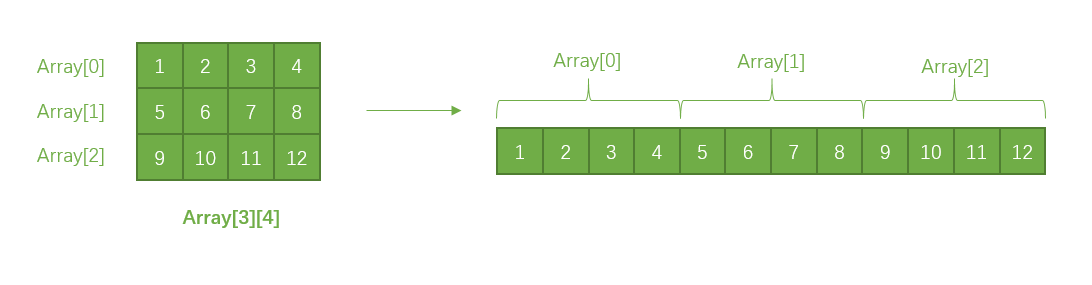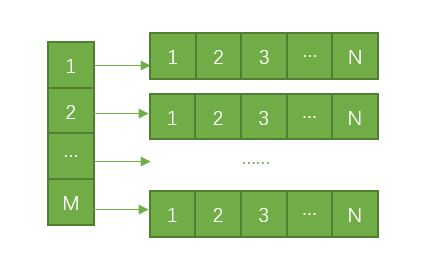# C/C++二维数组总结

7 篇文章 1 订阅

## 1、关于定义二维数组### 方法一： 直接确定二维数组的行和列数

/* 可以使用下面的方法来初始化二维数组
* 也就是直接将二维数组铺平，写成一维数组的形式
*/
int array = {1, 2, 3, 4, 5, 6, 7, 8, 9, 10, 11, 12};

/* 可以使用标准的二维数组的初始化方式
*/
int array = {{1, 2, 3, 4}, {5, 6, 7, 8}, {9, 10, 11, 12}};

### 方法二： 不用指明二维数组的行数，但要指明列数

/* 经典的写法
*/
int array[];

/* 一种更帅的写法，本质上是定义了一个数组指针
*/
int (*array);

### 方法三： 动态分配的方式，这种写法比较灵活，在定义的时候不需要指明二维矩阵的行数和列数

/* 使用动态分配内存的形式，用malloc
*/
int **array // array[M][N]
array = (int **)malloc(M * sizeof(int *));
for(int i = 0; i < M; i++) {
array[i] = (int *)malloc(N * sizeof(int));
}

// 释放
for(int i = 0; i < M; i++)
free(array[i]);
free(array);

/* 使用动态分配内存的形式，用new
*/
int **array // array[M][N]
array = new int*[M];
for(int i = 0; i < M; i++) {
array[i] = new int[N];
}

// 释放
for(int i = 0; i < M; i++)
delete [] array[i];
delete [] array;## 2 、二维数组的传参写法

### 1 使用动态分配内存的方式申请空间，可以使用**传递参数

#include <iostream>
using namespace std;

int func1(int **arr) {
cout << arr;
arr = 20;
return 0;
}

int main()
{
int **array
int row = 3;
int col = 4;
array = (int **)malloc(row * sizeof(int *));
for (int i = 0; i < row; i++) {
array[i] = (int *)malloc(col * sizeof(int));
}
memset(*array, 0, sizeof(int)*row*col);

array = 100;
func1(array);
cout << array;
return 0;
}

### 2 使用二维数组的传统定义方法传递参数

#include <iostream>
using namespace std;

/*
* 以下两种写法本质上是一样的
*/
int func1(int (*arr)) {
cout << arr << endl;
arr = 20;
return 0;
}

int func2(int arr[]) {
cout << arr << endl;
arr = 20;
return 0;
}

int main()
{
int array = {1,2,3,4,5,6,7,8,9,10,11,12};

array = 100;
func1(array);
cout << array << endl;
return 0;
}

template<class T>
int GetArrayLen(T& a)
{
return sizeof(a)/sizeof(a);
}

04-1908-148万+
04-242759
03-074489
04-282万+
09-04720
06-241万+
09-304万+
03-1913万+
11-211812
11-163826
09-05953
12-292767
04-153万+
03-18
04-022762
08-042914
01-063319
07-072402
02-072万+点击重新获取扫码支付余额充值